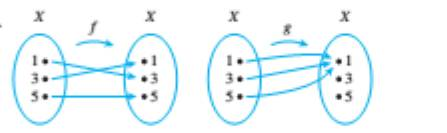Chapter 7.3, Problem 2ES### Discrete Mathematics With Applicat...

5th Edition
EPP + 1 other
ISBN: 9781337694193

#### Solutions

Chapter
Section### Discrete Mathematics With Applicat...

5th Edition
EPP + 1 other
ISBN: 9781337694193
Textbook Problem
1 views

# In each of 1 and 2, functions f and g are defined by arrow diagrams. Find g ∘ f and f ∘ g and determine g ∘ f equals f ∘ g .To determine

To find:

The values of gf and fg and check whether gf equals to fg.

Explanation

Given information:

Concept used:

gf=g(f(x))fg=f(g(x))

Calculation:

(gf)(1)=g(f(1))=g(3)=1(gf)(3)=g(f(3))=g(1)=1

Continuation of the above is,

(gf)(5)=g(f(5))=g(5)=1

Therefore,

gf={<

### Still sussing out bartleby?

Check out a sample textbook solution.

See a sample solution

#### The Solution to Your Study Problems

Bartleby provides explanations to thousands of textbook problems written by our experts, many with advanced degrees!

Get Started